# Definition of Orbitals

What are Orbitals?

Atomic orbitals describe where an electron is likely to be found in an atom. Molecular orbitals perform the same role in molecules.

Every atomic orbital is associated with three quantum numbers, n, l, and ml. These numbers:

• are obtained from the wave function Ψ
• describe the energy and angular momentum of the orbital's electron(s) and the orientation of the orbital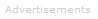The shape and size of an orbital can be determined from the square of the wave function Ψ2.

Atomic orbitals have distinctive shapes; all are centered on the atomic nucleus.

The most commonly encountered orbitals in elementary quantum chemistry are the orbitals corresponding to the s, p, and d subshells: these orbitals are named the s, p, and d orbitals. In the ground states of heavier atoms f orbitals are also encountered.

The order in which atomic orbitals are filled by electrons and the shapes of the orbitals are crucial factors in understanding the chemical behavior of atoms and their reactions.

s Orbitals

All s orbitals are spherical and can hold up to two electrons. The lowest energy orbital of all orbitals is the 1s orbital. When it holds one electron it is written 1s1; when it holds two electrons, it is written 1s2.

A typical s orbital is shown below: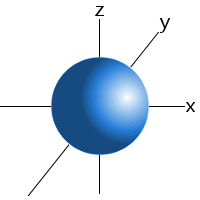An s orbital.

p Orbitals

The lowest energy p orbitals, the 2p, are shown below. They sit at right-angles to one another and are centered on the nucleus. Since their lengths can be aligned with x, y, and z axes, they are individually described as the px, py and pz orbitals.

pxpypzEach orbital can hold up to two electrons. The three p orbitals, therefore can accommodate up to six electrons. When one electron is accommodated by the 2p orbitals it is written 2p1; five electrons would be written 2p5.

Orbital Phase

The color differences in the p orbitals above represent different phases of the quantum mechanical wave function Ψ. Different phases are separate by nodes, where the electron density is zero.

Phase differences are important when, for example, the wave functions of two or more atoms combine to form a molecule or when two or more atomic orbitals hybridize. Wave functions with the same phase interfere constructively, leading to the possibility of bonding. Wave functions with different phases interfere destructively.

d Orbitals

The lowest energy d orbitals, the 3d, are shown below. There are five d orbitals, each of which can hold a maximum of two electrons, so in total, the d orbitals can accommodate up to ten electrons.

The d orbitals are:

dxy,   dxz,   dyz,   dx2-y2,   dz2

When one electron is accommodated by the 3d orbitals it is written 3d1; six electrons would be written 3d6; ten electrons would be written 3d10.

The d orbitals are named in relation to the x, y, and z axes. Once again, the different colors indicated different phases of the wave function.

dxy
This orbital is in the xy-plane. The four lobes do not touch the axes; they occupy the four quadrants between the x and y axes.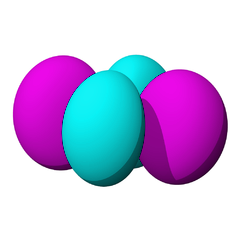dxz
This orbital is in the xz-plane. The four lobes do not touch the axes; they occupy the four quadrants between the x and z axes.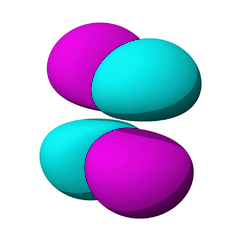dyz
This orbital is in the yz-plane. The four lobes do not touch the axes; they occupy the four quadrants between the y and z axes.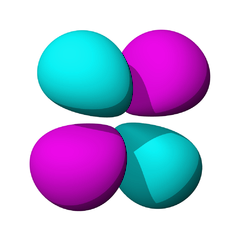dx2-y2
This orbital is in the xy-plane. The four lobes are aligned with the x and y axes.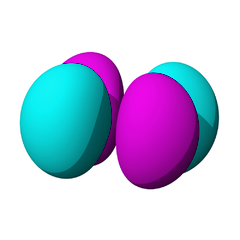dz2
This orbital is a bit like a p orbital with a Saturn-like-ring around its middle. This orbital's main lobes align with the z axis.For further details see sublevels, aufbau principle, and orbital hybridization.Search the Dictionary for More Terms